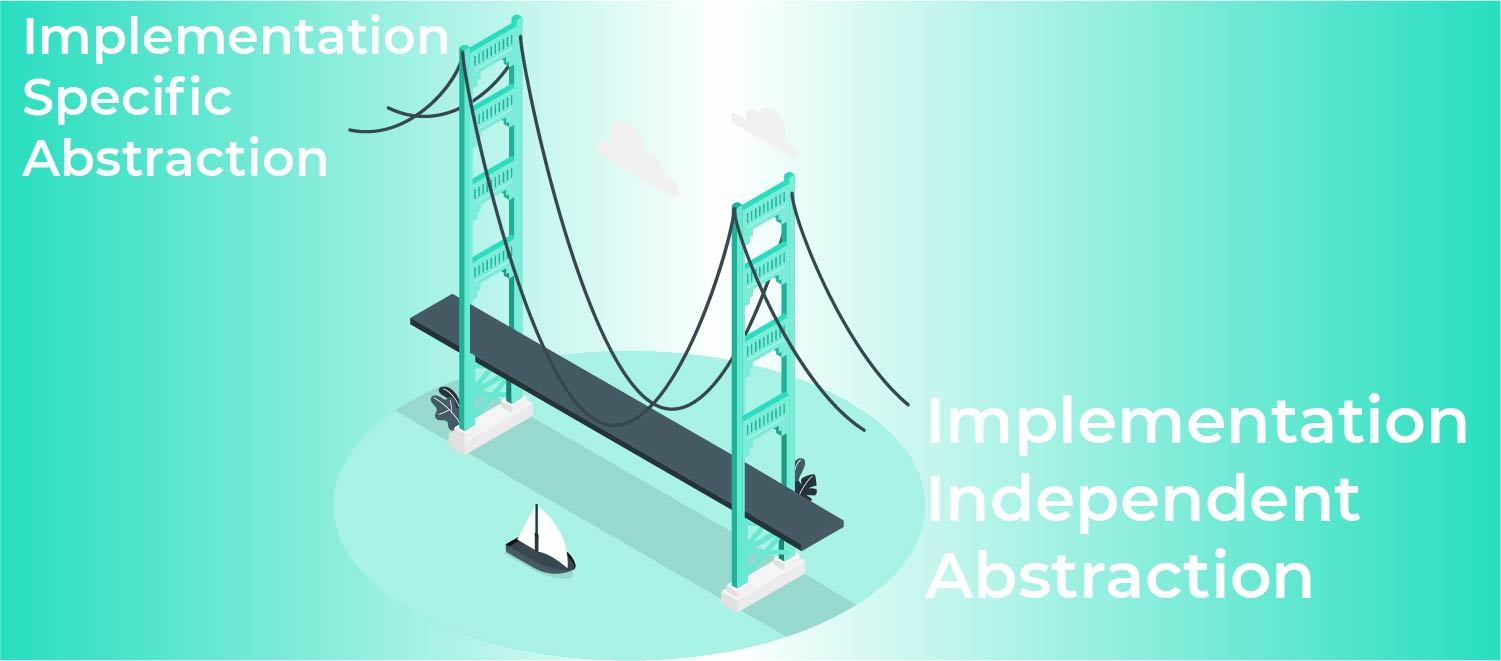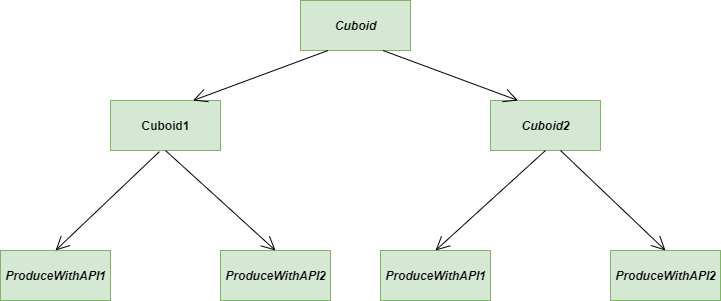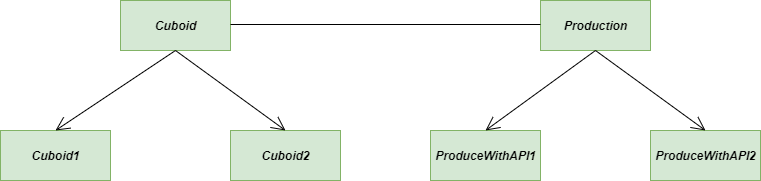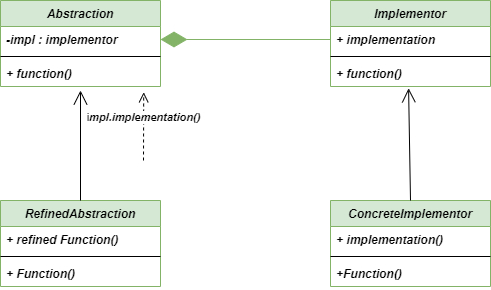# Bridge Method – Python Design Patterns

• Last Updated : 13 Jul, 2022

The bridge method is a Structural Design Pattern that allows us to separate the Implementation Specific Abstractions and Implementation Independent Abstractions from each other and can be developed considering as single entities.
The bridge Method is always considered as one of the best methods to organize the class hierarchy.Bridge Method

### Elements of Bridge Design Pattern

• Abstraction: It is the core of the Bridge Design Pattern and it provides the reference to the implementer.
• Refined Abstraction: It extends the abstraction to a new level where it takes the finer details one level above and hides the finer element from the implementers.
• Implementer: It defines the interface for implementation classes. This interface does not need to correspond directly to the abstraction interface and can be very different.
• Concrete Implementation: Through the concrete implementation, it implements the above implementer.

### Problem without using Bridge Method

Consider the following class Cuboid which has three attributes named length, breadth, and height and three methods named ProducewithAPI1(), ProduceWithAPI2(), and expand()
Out of these, producing methods are implementation-specific as we have two production APIs, and one method i.e., expand() method is implementation-independent.

Till now we have only two implementation-specific methods and one implementation-independent method but when the quantity will rise (of course in a large-scale project) things will become messy for the developers to handle.Problem-Bridge-Method

Note: Following code is written without using the Bridge method.

## Python3

 `""" Code without using the bridge method``    ``We have a class with three attributes``    ``named as length, breadth, and height and``    ``three methods named as ProduceWithAPI1(),``    ``ProduceWithAPI2(), and expand(). Out of these``    ``producing methods are implementation-specific``    ``as we have two production APIs"""` `class` `Cuboid:` `    ``class` `ProducingAPI1:` `        ``"""Implementation Specific Implementation"""` `        ``def` `produceCuboid(``self``, length, breadth, height):` `            ``print``(f``'API1 is producing Cuboid with length = {length}, '``                  ``f``' Breadth = {breadth} and Height = {height}'``)` `    ``class` `ProducingAPI2:``        ``"""Implementation Specific Implementation"""` `        ``def` `produceCuboid(``self``, length, breadth, height):``            ``print``(f``'API2 is producing Cuboid with length = {length}, '``                  ``f``' Breadth = {breadth} and Height = {height}'``)`  `    ``def` `__init__(``self``, length, breadth, height):` `        ``"""Initialize the necessary attributes"""` `        ``self``._length ``=` `length``        ``self``._breadth ``=` `breadth``        ``self``._height ``=` `height` `    ``def` `produceWithAPI1(``self``):` `        ``"""Implementation specific Abstraction"""` `        ``objectAPIone ``=` `self``.ProducingAPI1()``        ``objectAPIone.produceCuboid(``self``._length, ``self``._breadth, ``self``._height)` `    ``def` `producewithAPI2(``self``):` `        ``"""Implementation specific Abstraction"""` `        ``objectAPItwo ``=` `self``.ProducingAPI2()``        ``objectAPItwo.produceCuboid(``self``._length, ``self``._breadth, ``self``._height)` `    ``def` `expand(``self``, times):` `        ``"""Implementation independent Abstraction"""` `        ``self``._length ``=` `self``._length ``*` `times``        ``self``._breadth ``=` `self``._breadth ``*` `times``        ``self``._height ``=` `self``._height ``*` `times` `# Instantiate a Cubiod``cuboid1 ``=` `Cuboid(``1``, ``2``, ``3``)` `# Draw it using APIone``cuboid1.produceWithAPI1()` `# Instantiate another Cuboid``cuboid2 ``=` `Cuboid(``19``, ``20``, ``21``)` `# Draw it using APItwo``cuboid2.producewithAPI2()`

### Solution using Bridge method

Now let’s look at the solution for the above problem. The bridge Method is one of the best solutions for such kinds of problems. Our main purpose is to separate the codes of implementation-specific abstractions and implementation-independent abstractions.Solution-Bridge-Method

Note: Following Code is written using Bridge Method

## Python3

 `"""Code implemented with Bridge Method.``   ``We have a Cuboid class having three attributes``   ``named as length, breadth, and height and three``   ``methods named as produceWithAPIOne(), produceWithAPItwo(),``   ``and expand(). Our purpose is to separate out implementation``   ``specific abstraction from implementation-independent``   ``abstraction"""` `class` `ProducingAPI1:` `    ``"""Implementation specific Abstraction"""` `    ``def` `produceCuboid(``self``, length, breadth, height):` `        ``print``(f``'API1 is producing Cuboid with length = {length}, '``              ``f``' Breadth = {breadth} and Height = {height}'``)` `class` `ProducingAPI2:` `    ``"""Implementation specific Abstraction"""` `    ``def` `produceCuboid(``self``, length, breadth, height):` `        ``print``(f``'API2 is producing Cuboid with length = {length}, '``              ``f``' Breadth = {breadth} and Height = {height}'``)` `class` `Cuboid:` `    ``def` `__init__(``self``, length, breadth, height, producingAPI):` `        ``"""Initialize the necessary attributes``           ``Implementation independent Abstraction"""` `        ``self``._length ``=` `length``        ``self``._breadth ``=` `breadth``        ``self``._height ``=` `height` `        ``self``._producingAPI ``=` `producingAPI` `    ``def` `produce(``self``):` `        ``"""Implementation specific Abstraction"""` `        ``self``._producingAPI.produceCuboid(``self``._length, ``self``._breadth, ``self``._height)` `    ``def` `expand(``self``, times):` `        ``"""Implementation independent Abstraction"""` `        ``self``._length ``=` `self``._length ``*` `times``        ``self``._breadth ``=` `self``._breadth ``*` `times``        ``self``._height ``=` `self``._height ``*` `times`  `"""Instantiate a cuboid and pass to it an``   ``object of ProducingAPIone"""` `cuboid1 ``=` `Cuboid(``1``, ``2``, ``3``, ProducingAPI1())``cuboid1.produce()` `cuboid2 ``=` `Cuboid(``19``, ``19``, ``19``, ProducingAPI2())``cuboid2.produce()`

### UML Diagram of Bridge Method

Following is the UML diagram for Bridge MethodUML -diagram-Bridge-Method

• Single Responsibility Principle: The bridge method clearly follows the Single Responsibility principle as it decouples an abstraction from its implementation so that the two can vary independently.
• Open/Closed Principle: It does not violate the Open/Closed principle because at any time we can introduce the new abstractions and implementations independently from each other
• Platform independent feature: Bridge Method can be easily used for implementing the platform-independent features.

• Complexity: Our code might become complex after applying the Bridge method because we are intruding on new abstraction classes and interfaces.
• Double Indirection: The bridge method might have a slight negative impact on the performance because the abstraction needs to pass messages along with the implementation for the operation to get executed.
• Interfaces with only a single implementation: If we have only limited interfaces, then it doesn’t sweat a breath but if you have an exploded set of interfaces with minimal or only one implementation it becomes hard to manage

### Applicability

• Run-time Binding: Generally Bridge method is used to provide the run-time binding of the implementation, here run-time binding refers to what we can call a method at run-time instead of compile-time.
• Mapping classes: The bridge method is used to map the orthogonal class hierarchies
• UI Environment: A real-life application of the Bridge method is used in the definition of shapes in a UI Environment

Further read: Bridge Method in Java

My Personal Notes arrow_drop_up